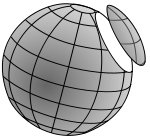xSmall circleEncyclopediaA circle of a sphere is a circle defined as the intersection of a sphere
Sphere
A sphere is a perfectly round geometrical object in three-dimensional space, such as the shape of a round ball. Like a circle in two dimensions, a perfect sphere is completely symmetrical around its center, with all points on the surface lying the same distance r from the center point...

and a plane
Plane
-Physical objects:* Aeroplane or airplane, a fixed-wing aircraft* Plane , a woodworking tool to smooth surfaces* Platanus, a genus of trees with the common name "plane"* Acer pseudoplatanus, a tree species sometimes called "plane"...

. If the plane contains the center of the sphere then the circle is called a great circle
Great circle
A great circle, also known as a Riemannian circle, of a sphere is the intersection of the sphere and a plane which passes through the center point of the sphere, as opposed to a general circle of a sphere where the plane is not required to pass through the center...

, otherwise it is a small circle. Circles of a sphere have radius less than or equal to the radius sphere, with equality when the circle is a great circle.

In the geographic coordinate system
Geographic coordinate system
A geographic coordinate system is a coordinate system that enables every location on the Earth to be specified by a set of numbers. The coordinates are often chosen such that one of the numbers represent vertical position, and two or three of the numbers represent horizontal position...

on a globe, the parallels of latitude
Latitude
In geography, the latitude of a location on the Earth is the angular distance of that location south or north of the Equator. The latitude is an angle, and is usually measured in degrees . The equator has a latitude of 0°, the North pole has a latitude of 90° north , and the South pole has a...

are such circles, with the Equator
Equator
An equator is the intersection of a sphere's surface with the plane perpendicular to the sphere's axis of rotation and containing the sphere's center of mass....

the only great circle. By contrast, all meridians of longitude
Longitude
Longitude is a geographic coordinate that specifies the east-west position of a point on the Earth's surface. It is an angular measurement, usually expressed in degrees, minutes and seconds, and denoted by the Greek letter lambda ....

, paired with their opposite meridian in the other hemisphere
Hemisphere
Hemisphere may refer to:*Half of a sphereAs half of the Earth:*Any half of the Earth, see Hemispheres of the Earth, see:...

, form great circles.

The diameter of the sphere when passes though the center of the circle is called its axis and the endpoints of this diameter are called its poles. A circle of a sphere can also be defined as the set of points at a given angular distance
Angular distance
In mathematics and all natural sciences , the angular distance between two point objects, as observed from a location different from either of these objects, is the size of the angle between the two directions originating from...

from a given pole.

## Geometric proof

That the intersection of a sphere and a plane is, in fact, a circle can be seen as follows. Let the S be a sphere with center O, P a plane which intersects S. Draw perpendicular to P and meeting P at E. , Let A and B be any two points in the intersection. Then AOE and BOE are right triangles with a common side, OE, and hypotenuses AO and BO, equal. Therefore the remaining sides AE and BE are equal. This proves that all points in the intersection are the same distance from the point E in the plane P, in other words all points in the intersection lie on a circle with center E. Note that OE is the axis of the circle.

As a corollary, on a sphere there is exactly one circle that can be drawn though three given points.

The proof can be extended to show that the points on a circle are all a common angular distance from one of its poles.